# Conversion of measurement units

Here you will learn about the conversion of units including conversions between metric units and between customary units.

Students will first learn about conversion of measurement as part of measurement and data in 5th grade.

## What is the conversion of measurement units?

The conversion of measurement units is taking a given unit, such as a length, capacity, mass or time and showing the same measurement with a different unit.

For example,

How many minutes are in 2 hours?

Hours is a measurement of time. Other measurements of time are seconds, minutes, weeks, years, decades, centuries,…

Using the relationship between each, you can convert between the units of time.

Since there are 60 minutes in 1 hour, there are 120 minutes in 2 hours.

\quad 60 minutes ( 1 hour)

+ \;\, \underline{60} minutes ( \underline{1} hour)

\;\; 120 minutes ( 2 hours)

Each measurement system has their own conversion factors.

• The metric system is based on powers of 10. It is considered the International system of units (SI) - SI units. The prefixes that come before the SI base units, such as kilo, centi, deci and milli, are used to indicate which power of 10 is involved.

For example,

\begin{aligned}& 1 \mathrm{~km}=1000 \mathrm{~m} \\\\ & 1 \mathrm{~m}=100 \mathrm{~cm} \\\\ & 1\mathrm{~kg}=1000 \mathrm{~g} \\\\ & 1 \mathrm{~cl}=10 \mathrm{~ml}\end{aligned}

• The customary system is an older system of measurements, but it is still used in some places. The customary units are NOT based on powers of 10.

For example,

\begin{aligned}& 1 \text { foot }=12 \text { inches } \\\\ & 3 \text { feet }=1 \text { yard } \\\\ & 1,760 \text { yards }=1 \text { mile }\end{aligned}

For both systems, you can convert units of measurement using conversion factors.

The conversion factor is a number you multiply or divide by to change units.

For example,

Below are the conversion factors for meters.

Convert 400 centimeters into meters.

There are 100 \, cm in 1 \, m. This is the conversion factor. To convert from cm to m you need to divide.

So, 400 \mathrm{~cm} \div 100=4 \mathrm{~m}.

For example,

Below are the conversion factors for capacity (volume) in the customary system.

Convert 9 quarts into pints.

There are 2 \text { pints } in 1 \text { quart }. This is the conversion factor. To convert from quarts to pints , you need to multiply.

So, 9 \text { quarts } \times 2=18 \text { pints }.

You can also use unit conversions for time.

For example,

How many minutes are there in 5 hours?

There are 60 minutes in 1 hour.

So, 5 \text { hours } \times 60=300 \text { minutes }.

### What is the conversion of measurement units?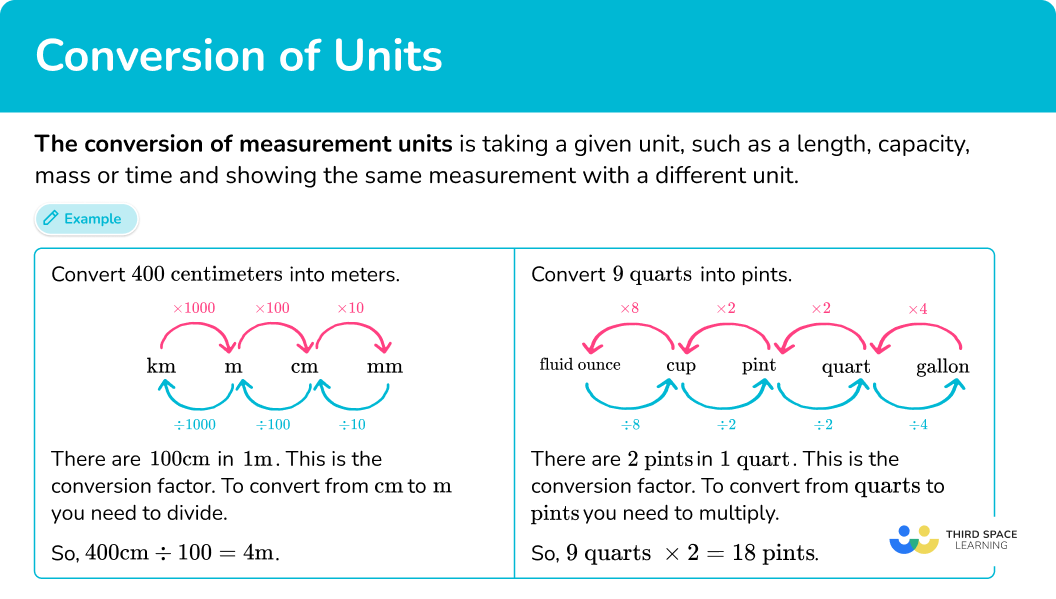## Common Core State Standards

How does this relate to 5 th grade math?

• Grade 5 – Measurement and Data (5.MD.A.1)
Convert among different-sized standard measurement units within a given measurement system (For example, convert 5 \, cm to 0.05 \, m ), and use these conversions in solving multi-step, real world problems.

## How to convert measurement units

In order to convert measurement units:

1. Find the conversion factor.
2. Multiply or divide by the conversion factor.

## Conversion of units examples

### Example 1: converting units of time

Convert 2 minutes to seconds.

1. Find the conversion factor.

Since seconds is the desired unit, you need the conversion factor between minutes and seconds:

1 \text { minute }=60 \text { seconds }

The conversion factor for minutes and seconds is 60.

2Multiply or divide by the conversion factor.

Look at the relationship in the original conversion.

From minutes to seconds, the relationship is multiplying by 60.

Multiply 2 minutes by 60 :

2 \times 60=120 \text { seconds }

2 \text { minutes }=120 \text { seconds }

### Example 2: converting units of length

Convert 180 inches to feet.

Since feet is the desired unit, you need the conversion factor between inches and feet:

1 \text { foot }=12 \text { inches }

The conversion factor for inches and feet is 12.

Look at the relationship in the original conversion.

From inches to feet, the relationship is dividing by 12.

Divide 180 inches by 12 :

180 \div 12=15 \text { feet }

180 \text { inches }= 15 \text { feet }

### Example 3: converting units of capacity

Convert 2.5 liters to milliliters.

Since milliliters is the desired unit, you need the conversion factor between liters and milliliters:

1 \text { liter }=1,000 \text { milliliters }

The conversion factor for liters and milliliters is 1,000.

Look at the relationship in the original conversion.

From liters to milliliters, the relationship is multiplying by 1,000.

Multiply 2.5 liters by 1,000 :

2.5 \times 1,000=2,500 \text { milliliters }

2.5 \text { liters }=2,500 \text { milliliters }

### Example 4: converting units of mass

Convert 7 kilograms to milligrams.

1 \text { kilogram }=1,000,000 \text { milligrams }

The conversion factor for kilograms to milligrams is 1,000,000.

Look at the relationship in the original conversion.

From kilograms to milligrams, the relationship is multiplying by 1,000,000.

Multiply 7 kilograms by 1,000,000 :

7 \times 1,000,000=7,000,000 \text { milligrams }

7 \text { kilograms }=7,000,000 \text { milligrams }

### Example 5: converting units of time

Convert 9,000 seconds to hours.

60 \text { seconds }=1 \text { minute } \quad and \quad 60 \text { minutes }= 1 \text { hour }

The conversion factor for minutes and seconds is 60 , and the conversion factor for minutes and hours is 60.

Look at the relationship in the original conversions.

From seconds to minutes, the relationship is dividing by 60.

Divide 9,000 seconds by 60 :

9,000 \div 60=150 \text { minutes }

From minutes to hours, the relationship is dividing by 60.

Divide 150 minutes by 60 :

150 \div 60=2.5 \text { hours }

9,000 \text { seconds }=2.5 \text { hours }

### Example 6: converting units of length

Convert 230 meters to kilometers.

1 \text { kilometer }=1,000 \text { meters }

The conversion factor for meters and kilometers is 1,000.

Look at the relationship in the original conversion.

From meters to kilometers, the relationship is dividing by 1,000.

Divide 230 meters by 1,000 :

230 \div 1,000=0.23 \text { kilometers }

230 \text { meters }=0.23 \text { kilometers }

### Teaching tips for conversion of measurement units

• Worksheets with a conversion table are a good way for students to practice converting measurements. Providing the table allows students to focus on the relationship instead of trying to remember the conversion rates.

• Give students opportunities to measure items around the classroom or school. This includes having them measure the same item in different units (like a desk in mm, cm and m ) or an amount of water in ml and l. This helps students understand that converting measurements doesn’t change the actual amount, it just changes the way it is measured.

• If students are really struggling, let them use a conversion calculator or unit converter, but require them to explain the conversion and the calculations that were made. With time, once they have seen enough examples, they can begin to solve problems without the additional assistance.

### Easy mistakes to make

• Fractions of an hour
Remember that time is not base 10. A quarter as a fraction is \cfrac{1}{4} and as a decimal is 0.25, but a quarter of an hour is 15 minutes.
For example,
3.5 hours is 3 hours and 30 minutes.
1 \cfrac{1}{2} days is 1 day and 12 hours.

• Confusing when to multiply and divide
Converting from a larger unit to a smaller unit (like meter to centimeter) is done by multiplying by 100. This can be confusing since going from larger to small units is done by multiplying. Always stop and think about the conversion before deciding which operation to use.

• Using the conversion factor to add or subtract
The relationship between units is multiplicative, which is why conversions are done in multiplication and division. Using addition or subtraction will lead to the incorrect answer.

• Thinking the conversion factor of area is the one dimensional factor
Area is a two-dimensional measurement and therefore does not convert in the same way as one-dimensional measurements.
For example,
1 \text { meter }=100 \text { centimeters}, but 1 \text { square meter } 100 \text { square centimeters }
1 \text { yard }=3 \text { feet }, but 1 \text { square yard } 3 \text { square feet }

• Thinking the conversion factor of volume is the one dimensional factor
Volume is a three-dimensional measurement and therefore does not convert in the same way as one-dimensional measurements.
For example,
1 \text { meter }=100 \text { centimeters}, but 1 \text { cubic meter } 100 \text { cubic centimeters }
1\text { foot }=12 \text { inches }, but 1 \text { cubic foot } 12 \text { cubic inches }

### Practice conversion of units questions

1. Convert 4.5 \, minutes to seconds\text{:}

270 \text { seconds }0.074 \text { seconds }27 \text { seconds }290 \text { seconds }The conversion factor between \text{minutes} and \text{seconds:}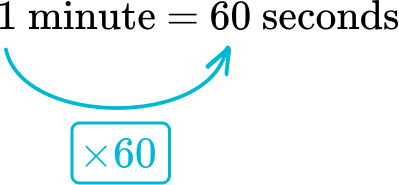Multiply 4.5 \text{ minutes} by 60 :

4.5 \times 60=270 \text { seconds }

4.5 \text { minutes }=270 \text { seconds }

2. Convert 144 \, \text{ounces} to pounds\text{:}

2,304 \text { pounds }12 \text { pounds }9 \text { pounds }14.4 \text { pounds }The conversion factor between \text{ounces} and \text{pounds:}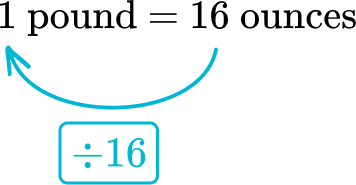Divide 144 \, minutes by 16 :

144 \div 16=9 \text { pounds }

144 \text { ounces }=9 \text { pounds }

3. Convert 3,400 \, km to m\text{:}

3.4 \mathrm{~m}340 \mathrm{~m}34,000 \mathrm{~m}3,400,000 \mathrm{~m}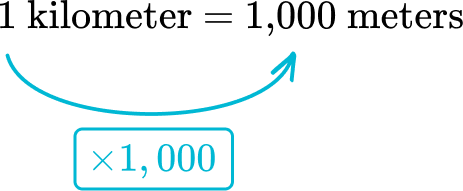Multiply 3,400 \, kilometers by 1,000 :

3,400 \times 1,000=3,400,000 \text { meters }

3,400 \text { kilometers }=3,400,000 \text { meters }

4. Convert 5 \, \text{gallons} to \text{cups:}

50 \text { cups }80 \text { cups }16 \text { cups }20 \text { cups }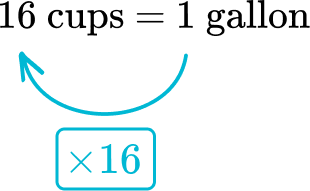Multiply 5 \, \text{gallons} by 16 :

5 \times 16=80 \, \mathrm{cups}

5 \text { gallons }=80 \text { cups }

5. Convert 2,560 \, \text{milliliters} to \text{liter:}

2. 56 \text { liters }25,600 \text { liters }256 \text { liters }0.256 \text { liters }Divide 2,560 \, \text{milliliters} by 1,000 :

2,560 \div 1,000=2.56 \text { liters }

2,560 \text { milliliters }=2.56 \text { liters }

6. Convert 5 \, \text{hours} to \text{seconds:}

300 \text { seconds }18,000 \text { seconds }50,000 \text { seconds }500 \text { seconds }The conversion factor between \text{hours} and \text{minutes:}Multiply 5 \, hours by 60 :

5 \times 60=300 \text { minutes }

The conversion factor between \text{minutes} and \text{seconds:}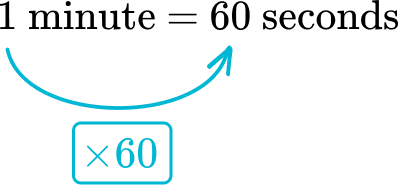Multiply 300 \, \text{minutes} by 60 :

300 \times 60=18,000 \text { seconds }

5 \text { hours }=18,000 \text { seconds }

## Conversion of measurement units FAQs

What is scientific notation?

It is a shorthand way to write very large or small numbers based on their power of 10.

What type of measurement is Fahrenheit?

Fahrenheit is a measurement of temperature. Celsius is another commonly used temperature measurement. The conversion between them is more complicated than the examples shown on this page. The formula used to convert between them is F=C \times \cfrac{9}{5}+32.

## Still stuck?

At Third Space Learning, we specialize in helping teachers and school leaders to provide personalized math support for more of their students through high-quality, online one-on-one math tutoring delivered by subject experts.

Each week, our tutors support thousands of students who are at risk of not meeting their grade-level expectations, and help accelerate their progress and boost their confidence.